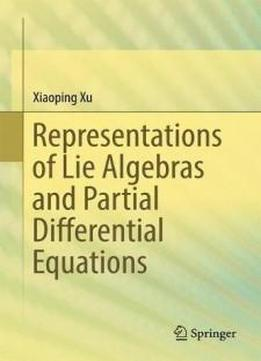# Representations Of Lie Algebras And Partial Differential Equations by Xiaoping Xu / 2017 / English / EPUB

This book provides explicit representations of finite-dimensional simple Lie algebras, related partial differential equations, linear orthogonal algebraic codes, combinatorics and algebraic varieties, summarizing the author’s works and his joint works with his former students.  Further, it presents various oscillator generalizations of the classical representation theorem on harmonic polynomials, and highlights new functors from the representation category of a simple Lie algebra to that of another simple Lie algebra.

This book provides explicit representations of finite-dimensional simple Lie algebras, related partial differential equations, linear orthogonal algebraic codes, combinatorics and algebraic varieties, summarizing the author’s works and his joint works with his former students.  Further, it presents various oscillator generalizations of the classical representation theorem on harmonic polynomials, and highlights new functors from the representation category of a simple Lie algebra to that of another simple Lie algebra. Partial differential equations play a key role in solving certain representation problems. The weight matrices of the minimal and adjoint representations over the simple Lie algebras of types E and F are proved to generate ternary orthogonal linear codes with large minimal distances. New multi-variable hypergeometric functions related to the root systems of simple Lie algebras are introduced in connection with quantum many-body systems in one dimension. In addition, the book identifies certain equivalent combinatorial properties on representation formulas, and the irreducibility of representations is proved directly related to algebraic varieties. The book offers a valuable reference guide for mathematicians and scientists alike. As it is largely self-contained – readers need only a minimal background in calculus and linear algebra – it can also be used as a textbook.

Partial differential equations play a key role in solving certain representation problems. The weight matrices of the minimal and adjoint representations over the simple Lie algebras of types E and F are proved to generate ternary orthogonal linear codes with large minimal distances. New multi-variable hypergeometric functions related to the root systems of simple Lie algebras are introduced in connection with quantum many-body systems in one dimension. In addition, the book identifies certain equivalent combinatorial properties on representation formulas, and the irreducibility of representations is proved directly related to algebraic varieties. The book offers a valuable reference guide for mathematicians and scientists alike. As it is largely self-contained – readers need only a minimal background in calculus and linear algebra – it can also be used as a textbook.

views: 672